# Array Abstraction

(diff) ← Older revision | Latest revision (diff) | Newer revision → (diff)

(in-progress)

Array abstraction is one of the most powerful features in Analytica. Although conceptually simple, Analytica modelers find that their mastery of array abstraction continues to improve over the course of years. Array abstraction provides many benefits:

• Flexibility: Easy to alter an index, e.g., adding or deleting elements.
• Hyper-flexibility: Easy to adjust the dimensionality of a model, even late in the modeling process.
• Direct what-if analysis and parametric analysis.
• Simplifies expressions, which increases transparency
• Reduces the cognitive load of the modeler, with dramatic productivity gains during model creation.
• Speed - Analytica is an array-based semi-interpreted language, but array operations, the bulk of the computation, occur in "native code".
• Representational Power: Any simple scalar function becomes a powerful array function when abstracted.
• Synergy with probabilistic inference: Monte Carlo and Latin Hypercube simulation are accomplished in Analytica through array abstraction. The Run index (i.e., the simulation index), is just another dimension, and the propagation of uncertainties through the model is an instance of array abstraction at work.

## Array abstraction of a scalar function

### One parameter function

The function Sqrt(x) computes the square root of «x». When you supply an array as a parameter, array abstraction iterates the function over all elements of the array and returns an array with the same dimensionality as the original.

A :=Sqrt(A) :=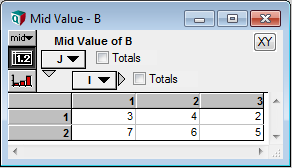Sqrt is an example of a scalar function with one parameter. We say that it treats its parameter as atomic, or it treats its parameter as an atom.

### Parameters have same indexes

The plus operator treats both its parameters as atoms. If both values supplied to the parameters have the same dimensionality, then array abstraction iterates over each index combination, producing an array result with the same dimensionality as the parameters.

Variable B := Sqrt(A)
A + B →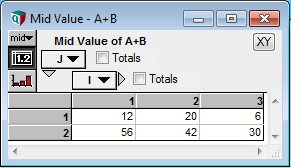### An array parameter with a scalar parameter

The Mod(x, y) function returns the remainder of dividing «x» by «y». It treats both parameters as atoms. When one parameter is an array and the other is scalar, array abstraction iterates over each cell of the array and applies the function using the same scalar value.

Mod(A, 5) →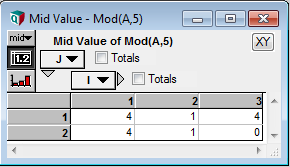### Equivalence of a scalar and a non-varying array

When you combine an array with a scalar, you get the same result as you would get if you combined the array with another array having the same indexes, but where every cell contains the scalar value.+ 5 →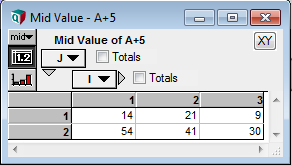+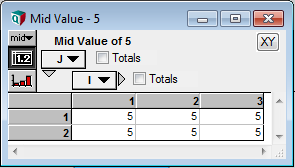→We say that an array that does not vary is equivalent to scalar with respect to array abstraction. It is a general principle that exchanging a value with an equivalent value in a computation produces an equivalent result. The indexes of the new result might be different, but if so, the result will be constant along any index that does not appear in its equivalent result.

This principle of equivalence gives rise to a general principle in Analytica modeling: You only need to include an index if the data you are representing varies over that index.

An inverse perspective is that you can conceptualize any value has if it has every index, but does not vary along those indexes that aren't shown.

### Combining arrays with different indexes

When the two (or more) parameters to a scalar function or operator are arrays with different indexes, the result has the union of the indexes from the parameters. For example, if the first parameter has indexes I and J, and the second parameter has indexes J and K, the result has indexes I, J, and K.

From the previous subsection, we saw that an array index by I and J is equivalent to an array indexed by I, J, and K which does not vary along K. Similarly, an array indexed by J and K is equivalent to and array indexed by I, J, and K that does not vary along I. You can substitute these equivalent arrays for the originals to obtain two arrays with identical dimensionality, which is a case covered above.

Another way to think about it is that for each cell in the result, find the cell in each parameter that matches on the coordinates for the indexes that the result and parameter has in common. Apply the scalar function to those cells to obtain the result.

Consider this example:+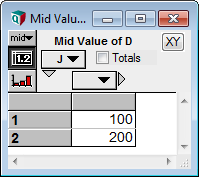→First, observe that the result has all indexes from the parameters. Then, notice that the result cell for [I = 3, J = 2] is C[I = 3] + D[J = 2]. You should study the example to understand where each result cell comes from. You should also study the next example.+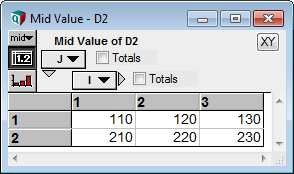→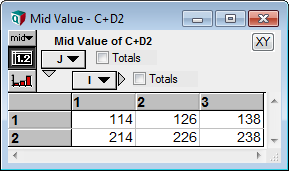### Test yourself

The two indexes named Tens and Ones are defined as 0..9, and Pos has these indexes as shown.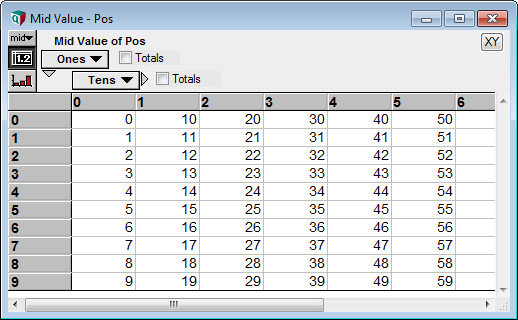• Write a short expression for the Definition of Pos that produces this result. Hint: It requires only two arithmetic operations.

The array N_sqr has index N, N := 0..99, and each cell is equal to the square of N.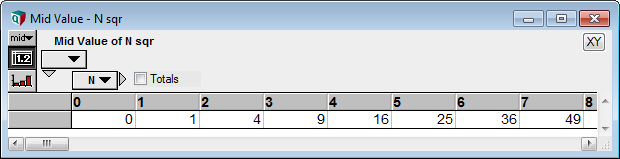The Subscript expression, N_sqr[N = Pos], can be viewed as a scalar function of Pos. In function syntax, this can be equivalently written as Subscript(N_sqr, N, Pos). Note: The first parameter of Subscript is not a scalar -- it expects an array, but here you can visualize that as being fixed, so that we are essentially considering the function

Function F(x) := N_sqrt[N = x]

which is a scalar function of x.

• Use the principles of array abstraction on scalar functions to find the result of N_sqrt[N = Pos]. Note: An array, Pos is being passed to a parameter that is treated as an atom.

## Array abstraction of a function with an array parameter

The above section considered the case where a function treats a parameter as atomic. Let's now consider the case where a function operates over an array.

### Functions with one array parameter

The function Sum(x, I) expects an array for the first parameter. It also expects an index as a second parameter, which tells the function which index to operate over. It is generally the case for a functions that array-abstracts that an array parameter is accompanied by an index (or indexes) specified which indexes are operated over. You can say that Sum expects the first parameter to be an array indexed by «I». In other words, it expects the first parameter to be a one-dimensional array.

Although Sum(x, I) expects «x» to be one-dimensional, there is no problem with passing a 2-D, or even 10-D, value to the first parameter. Once again, array abstraction kicks in and iterates over all combinations the indexes of «x» excluding index «I». For each combination, it takes the slice that remains and applies the function.

A :=Sum(A, I) :=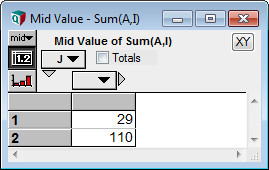In the example here, A contains and extra index, J. So Sum(A, I) iterates over J. It calculates the sum over I for the slice A[J = 1], and then again for the slice A[J = 2].

• Test yourself: Notice that Sum(A, I)[J = 2] is the same as Sum(A[J = 2], I). Is there a general principle here?